# Resizing examples

Example 1

Enlarge the following shape by a scale of 3 from the graph origin.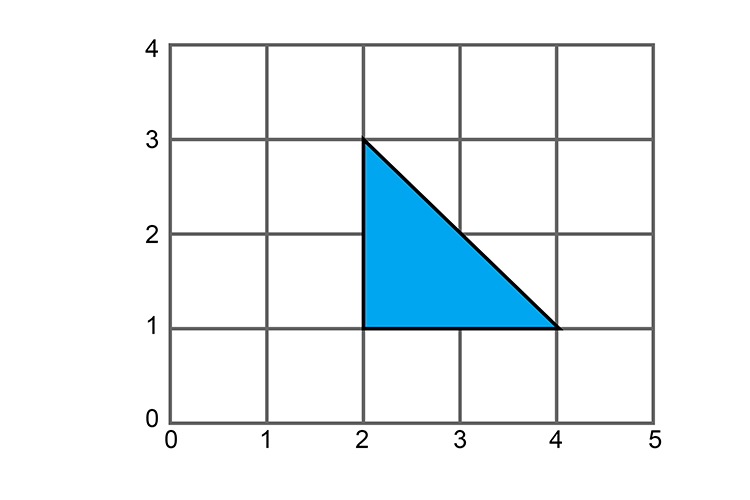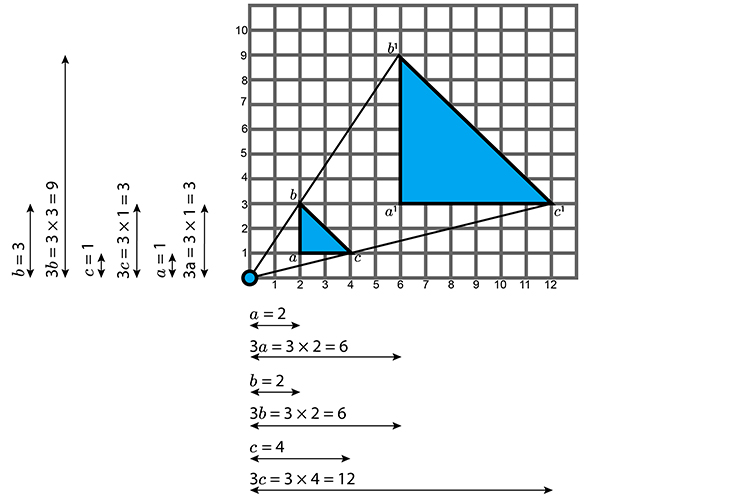Example 2

Resize the following shape by a 1/3 from the centre co-ordinates (1,1).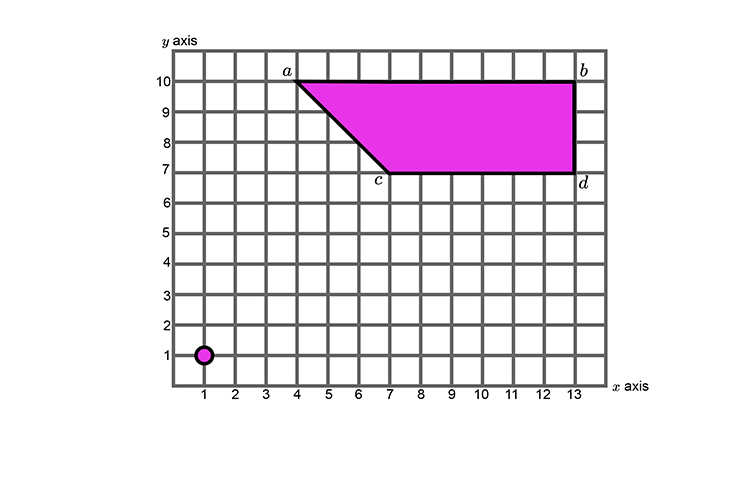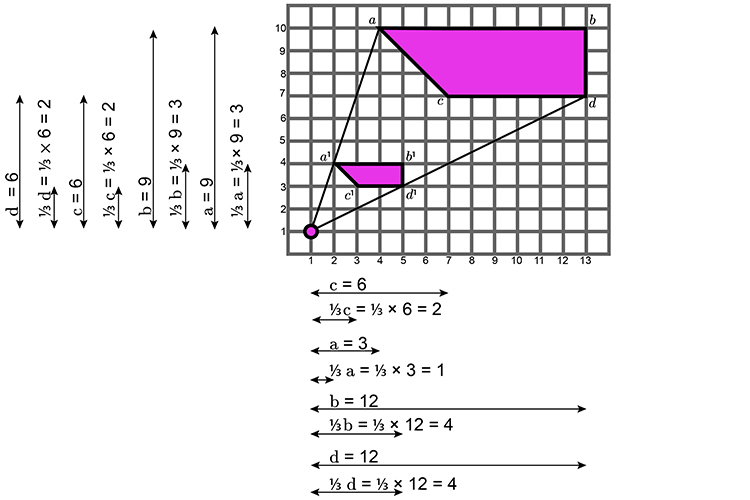Example 3

Resize the following rectangle by a factor of -2  with the centre at the origin (of the graph).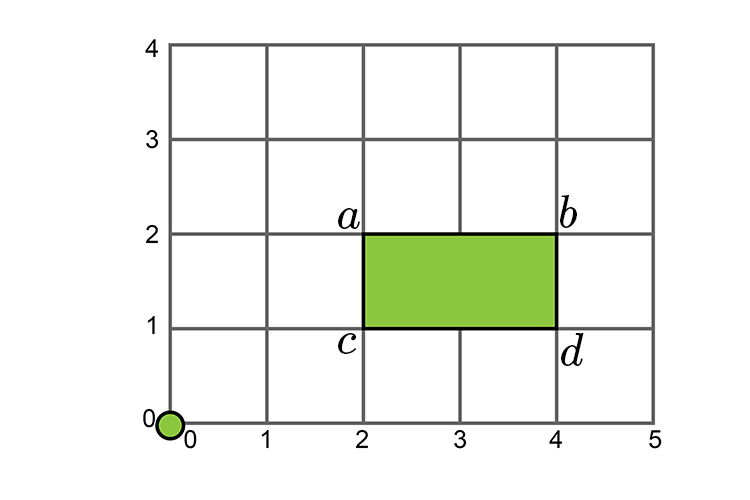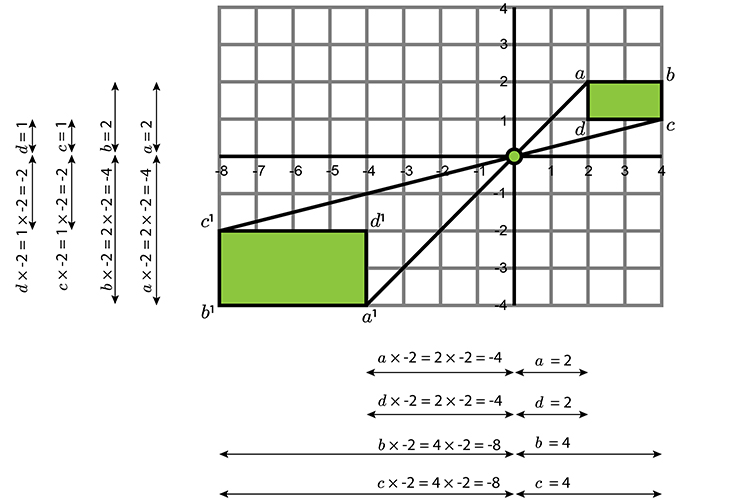Example 4

Enlarge the following triangle by a scale of 3 from the graph origin (0).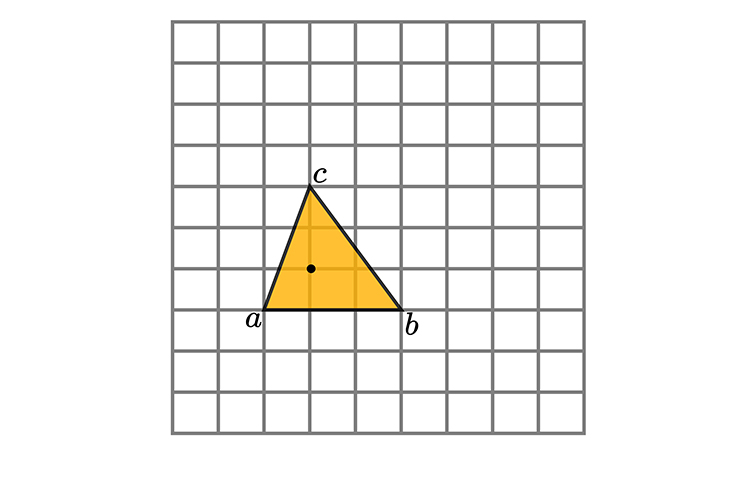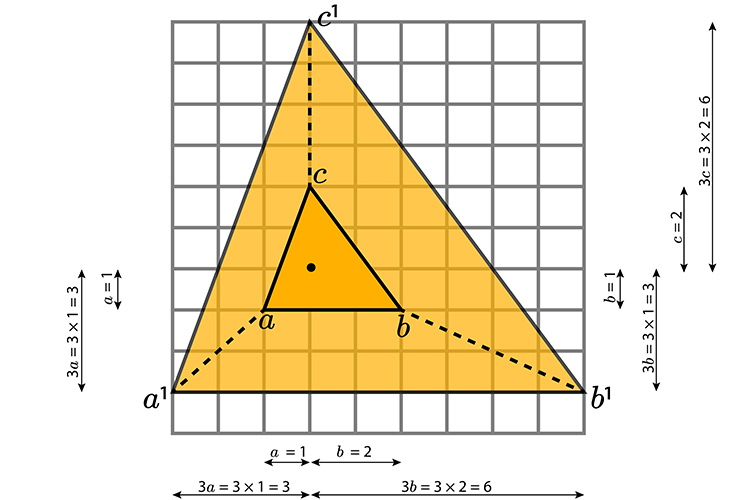Example 5

Describe fully the transformation which maps triangle A onto Triangle B.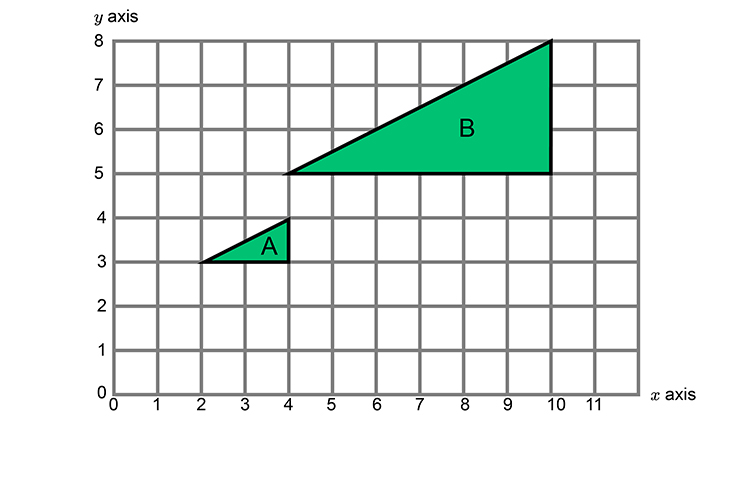The first stage is to find the origin which you can achieve by joining the respective corners with a line and extending them.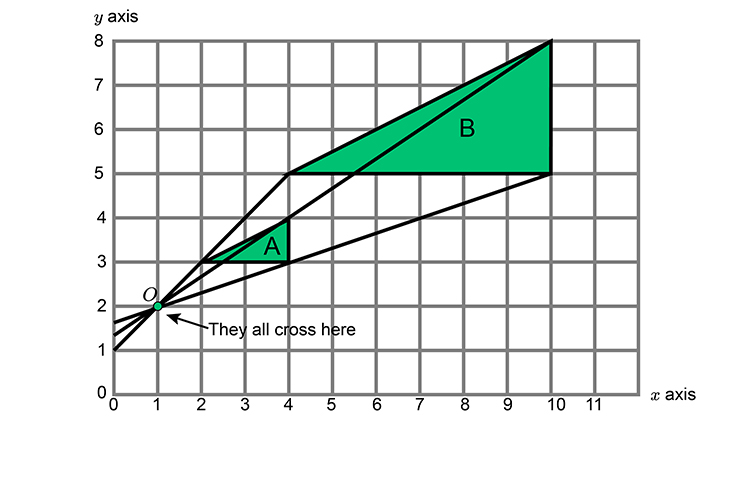The extended lines all cross at point O which must therefore be the CENTRE point.

We must now work out the scale.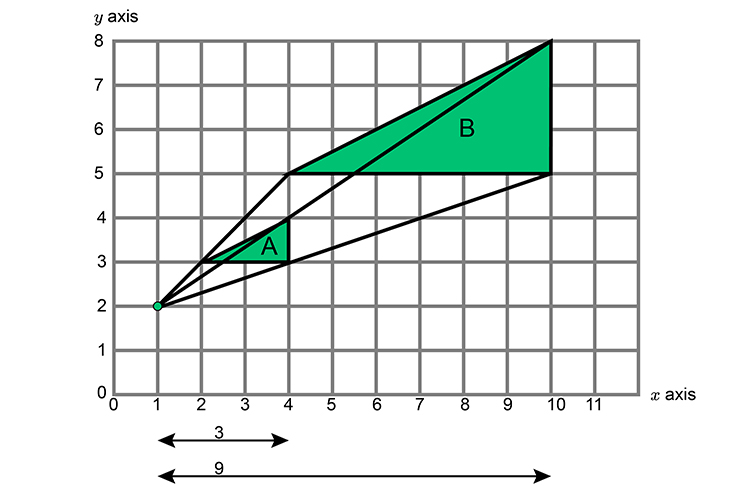Take any point and on one side alone work out the change in scale.

3  becomes 9

3timesx=9

x=9/3

x=3

## Check

3times3=9

So the answer is: The single transformation which maps triangle A onto triangle B is an enlargement with centre of coordinate (1,2) and scale of +3.